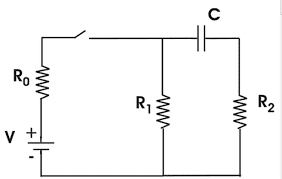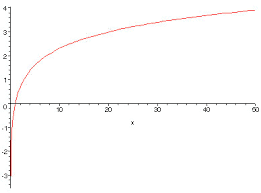# Graphing Current v. Time for simple 2R1C circuit

• friendbobbiny

#### friendbobbiny

1. Homework StatementThe switch is closed. Graph the current in $R_{1}$

2. Homework Equations

$V = IR$
$CV = Q$

3. The Attempt at a Solution

1) $R_{0}$ can be ignored
a) The resistor simply reduces the voltage before any current can be split.
b) We're justified, as a result, in imagining that $R_{0}$ were removed.

2) Through the branch with C and $R_{2}$, current will eventually stop flowing
a) Capacitor C will acquire a potential difference of V
b) When that happens, Kirchoff's loop rule -- that voltage drops and gains belonging to a circuit LOOP sum to zero -- won't need to be fulfilled by having current pass through $R_{2}$
c) When current doesn't pass through $R_{2}$, the branch can't be completed and current won't flow through it at all

d) Is this sound reasoning? Or, is it the case that current does continue flowing through the capacitor but just won't go any further?

3) If current doesn't enter the capacitor, more of it enters $R_{1}$, our resistor of interest
a) The current entering $R_{1}$ will change at the rate that current decreases the capacitor C.
b) Eventually, current approaches an asymptote: V/R

4) The resulting graph is logarithmic, except that it has an asymptote at y = $V/R$

Note! Do not rely on this graph. Just frames the idea.Last edited:
If R0 can be ignored (?) then the voltage across R1 would simply be the battery voltage, constant.

You show a graph. How did you determine that it should start at that negative potential?

Capacitor C will eventually acquire a potential difference of V? Only if R0 is zero.

Last edited:
If R0 can be ignored (?) then the voltage across R1 would simply be the battery voltage, constant.

Doesn't the current that would otherwise enter the cacpacitor enter the resistor?

As current going through the cacpacitor decreases, doesn't it increase through the resistor?

Doesn't the current that would otherwise enter the cacpacitor enter the resistor?

As current going through the cacpacitor decreases, doesn't it increase through the resistor?
Not really. The total current provided by the voltage source (and flowing through R0) will change over time. So your argument doesn't lead to concrete predictions. What if the initial source current is greater than the final current? Will the conjectured increase in proportion of the R1 current make up for the decrease in total current available at the "split"?

Approach the problem in the standard fashion.

Assume that the capacitor is initially uncharged (Vc = 0). At time t = 0 the switch closes. What does the capacitor look like at time t = 0+? So what does the circuit look like at t = 0+? What's the current in R1?

After a long time the circuit reaches steady state. What does the capacitor look like at steady state? So what does the circuit look like then? What is the current through R1 at steady state?

Check the current through R0 at t = 0+ and at steady state. Are they the same? How does that affect your argument about current splitting?

•friendbobbiny
Assume that the capacitor is initially uncharged (Vc = 0). At time t = 0 the switch closes. What does the capacitor look like at time t = 0+? So what does the circuit look like at t = 0+? What's the current in R1?

After a long time the circuit reaches steady state. What does the capacitor look like at steady state? So what does the circuit look like then? What is the current through R1 at steady state?

$V - I_{0}R_{0} - I_{1}R_{1} = 0$
$V - I_{0}R_{0} - Q/C - I_{2}R_{2} = 0$
$I_{0} = I_{1} + I_{2}$

So

$V - I_{0}R_{0} = I_{1}R_{1}$
Substitute this into Equation #2
and
$I_{1}R_{1} = Q/C + I_{2}R_{2}$

but Q = 0. So
$I_{1}R_{1} = I_{2}R_{2}$

The resistors are in parallel and can be resolved easily.

The outcome will $> 0$

Eventually, the branch through the capacitor will stop flowing, because the capacitor will be fully charged. (Just because it's fully charged (at the battery's voltage, consequently), why will current stop flowing?) I'm not convinced by an explanation I found:

That capacitor and battery can be treated as equipotential points -- so that charges between them have no reason to move anywhere. I'd reason that charges should continue along the low-resistance wire and then lose their energy upon going through the capacitor. Thus, charges should still flow but not continue.

When the capacitor's voltage = the battery's voltage, current will be constant and given by $V /(R_{0} + R_{1})$

$V - I_{0}R_{0} - I_{1}R_{1} = 0$
$V - I_{0}R_{0} - Q/C - I_{2}R_{2} = 0$
$I_{0} = I_{1} + I_{2}$

So

$V - I_{0}R_{0} = I_{1}R_{1}$
Substitute this into Equation #2
and
$I_{1}R_{1} = Q/C + I_{2}R_{2}$

but Q = 0. So
$I_{1}R_{1} = I_{2}R_{2}$

The resistors are in parallel and can be resolved easily.

The outcome will $> 0$
I was hoping more for an expression for the current through R1 at the instant t = 0+.
At the instant of the switch closure the capacitor will behave as a piece of wire. You should be able to find an expression for the current through R1.
Eventually, the branch through the capacitor will stop flowing, because the capacitor will be fully charged. (Just because it's fully charged (at the battery's voltage, consequently), why will current stop flowing?) I'm not convinced by an explanation I found:

That capacitor and battery can be treated as equipotential points -- so that charges between them have no reason to move anywhere. I'd reason that charges should continue along the low-resistance wire and then lose their energy upon going through the capacitor. Thus, charges should still flow but not continue.
The capacitor will NOT be charged to the battery's potential. There's a voltage divider between the battery and the capacitor branch.

Current will not flow "uphill" against a potential difference. Charges will never flow though a capacitor (unless there's a component failure). Charges can only accumulate on the plates or be removed from the plates. Current "through" a capacitor is the result of the electric field of the charges on one plate influencing the charges on the opposite plate. This results in potential differences across the capacitor.

Charges moving onto a capacitor plate increase the potential difference across the capacitor. Once the capacitor's potential difference reaches that of the source of the charges no further charges can flow to the capacitor --- they would be flowing "uphill" as any additional charge must increase the potential difference and the charge would be compelled to flow back "downhill". An equilibrium with no net current to or from the capacitor is established.

When the capacitor's voltage = the battery's voltage, current will be constant and given by $V /(R_{0} + R_{1})$[/QUOTE]
Again, the capacitor voltage cannot reach that of the source voltage under any time. The voltage divider comprised by R0 and R1 precludes this possibility. But your expression for the current at steady state is correct :)